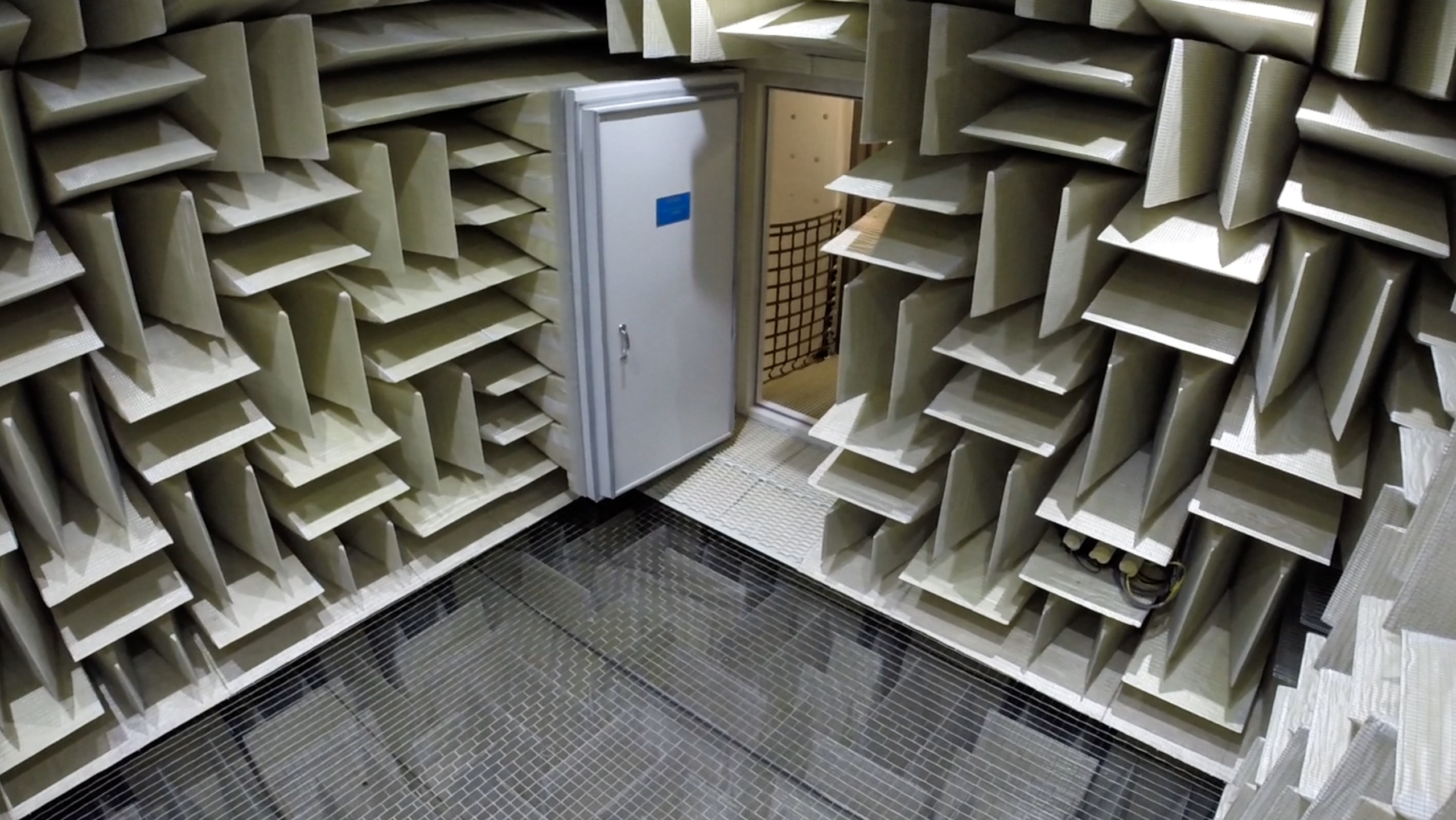## Sound Pressure vs Power

Explain the difference between sound pressure and sound power.Hint
The SI unit for sound power is watt ( $$W=J/s$$ ). The SI unit for sound pressure is pascal ( $$Pa=N/m^2$$ ). To generalize, sound power is the cause, and sound pressure is the effect.
Sound power is the total sound energy radiated by a source per unit of time. Sound pressure is a measurement (at a specific location) of a sound source as it is transfers throughout a special acoustical environment. In other words, sound power is the cause, and sound pressure is the effect.
Sound pressure is a measurement (at a specific location) of a sound source as it is transfers throughout a special acoustical environment. Sound Power is the total sound energy coming from a device per unit time.